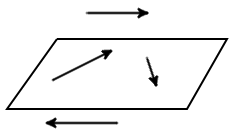Study of mathematics online.
Study math with us and make sure that "Mathematics is easy!"

# Coplanar vectors

Definition. Vectors parallel to the same plane, or lie on the same plane are called coplanar vectors (Fig. 1).Fig. 1

It is always possible to find a plane parallel to the two random vectors, in that any two vectors are always coplanar.

## Condition of vectors coplanarity

Example 1. Check whether the three vectors are coplanar a = {1; 2; 3}, b = {1; 1; 1}, c = {1; 2; 1}.

Solution: calculate a scalar triple product of vectors

 a · [b × с] = 1 2 3 = 1 1 1 1 2 1

= 1·1·1 + 1·1·2 + 1·2·3 - 1·1·3 - 1·1·2 - 1·1·2 = 1 + 2 + 6 - 3 - 2 - 2 = 2

Answer: vectors are not coplanar as their scalar triple product is not zero.

Example 2. Prove that the three vectors a = {1; 1; 1}, b = {1; 3; 1} и c = {2; 2; 2} are coplanar.

Solution: calculate a scalar triple product of vectors

 a · [b × с] = 1 1 1 = 1 3 1 2 2 2

= 1·2·3 + 1·1·2 + 1·1·2 - 1·2·3 - 1·1·2 - 1·1·2 = 6 + 2 + 2 - 6 - 2 - 2 = 0

Answer: vectors are coplanar as their scalar triple product is zero.

Example 3. Check whether the vectors are collinear a = {1; 1; 1}, b = {1; 2; 0}, c = {0; -1; 1}, d = {3; 3; 3}.

Solution: Find the number of linearly independent vectors, for this we write the values of the vectors in a matrix and run at her elementary transformations1 1 1~ 1 2 0 0 -1 1 3 3 3

from 2 row we subtract the 1-th row; from 4 row we subtract the 1-th row multiplied by 3;

 ~1 1 1~1 1 1~ 1 - 1 2 - 1 0 - 1 0 1 -1 0 -1 1 0 -1 1 3 - 3 3 - 3 3 - 3 0 0 0

for 3 row add 2 row

 ~1 1 1~1 1 10 1 -1 0 1 -1 0 + 0 -1 + 1 1 + (-1) 0 0 0 3 - 3 3 - 3 3 - 3 0 0 0

Since there are two non-zero row, then among the given vectors only two linearly independent vectors.

Answer: vectors are coplanar since there only two linearly independent vectors.